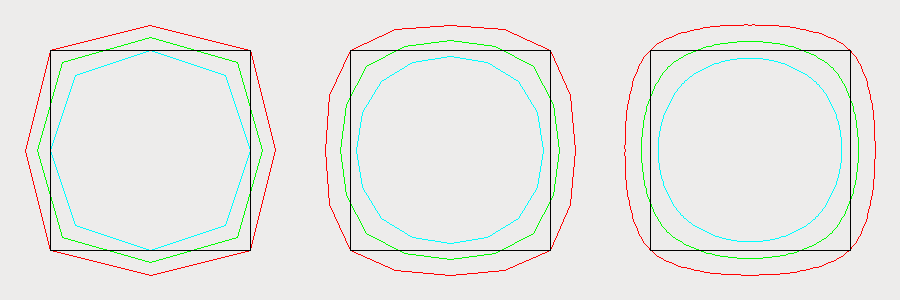++ed by:
1 non-PAUSE user

# NAME

Math::JSpline - Native perl extension for multi-dimensional J-Spline curves (open and closed)

# SYNOPSIS

``````  use Math::JSpline;
my(\$newx,\$newy)=&JSpline(\$subdivision_level, \$a, \$b, \$end_type, \@x, \@y);``````

where

``````  \$subdivision_level determines how many points to interpolate (1 doubles \$#x in the above example).
when \$a = \$b, this is the "s" paramater described below
\$end_type is how you want to deal with the start and end points.
3=join them up (loop). 2=tangent clamp, 1=end clamp, 0=simple join (refer "see also" below)
\@x... any number of array references come next``````

# DESCRIPTION

J-Splines are awesome: they're a class of spline curves that take a shape parameter, s. Setting s=1 yields uniform cubic B-spline curves, s=0 gives four-point subdivision curves, s=0.5 are uniform quintic B-splines - and more. "s" basically governs the "snappiness" of the curve.

Math::JSpline, lets you choose any combination of J-Spline parameters for any number of input arrays, with a range of different starting and ending schemes (eg: open or closed loops). Use it for 2D drawing, 3D graphics, or any other kind of interpolation you might need.

JSpline

## EXAMPLE

``````  #!perl -w
use Math::JSpline;
my (\$x)=&JSpline(1,0.5,0.5,3,[1,2,3,4]);
print join(" ",@{\$x});``````

returns

``  1.25 1.375 2 2.5 3 3.625 3.75 2.5 1.25``

## 2D Demo

The example source included in this distro produces the following output (see also: the js.pdf below)# SEE ALSO

http://faculty.cs.tamu.edu/schaefer/research/js.pdf

# AUTHOR

Chris Drake, <cdrake@cpan.org>

# COPYRIGHT AND LICENSE

Copyright (C) 2013 by Chris Drake

This library is free software; you can redistribute it and/or modify it under the same terms as Perl itself, either Perl version 5.10.1 or, at your option, any later version of Perl 5 you may have available.